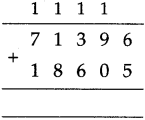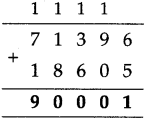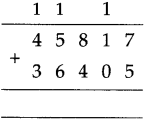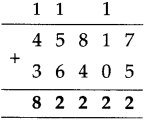# Maharashtra Board Class 5 Maths Solutions Chapter 3 Addition and Subtraction Problem Set 7

Balbharti Maharashtra Board Class 5 Maths Solutions Chapter 3 Addition and Subtraction Problem Set 7 Textbook Exercise Important Questions and Answers.

## Maharashtra State Board Class 5 Maths Solutions Chapter 3 Addition and Subtraction Problem Set 7

Question 1.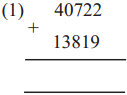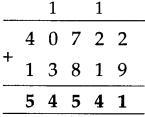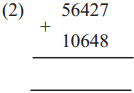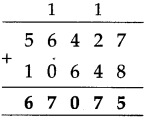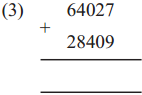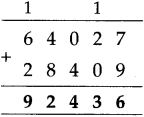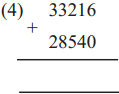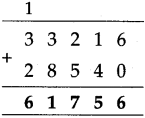Addition of six-digit and seven-digit numbers

Last year, you have learned to add five-digit numbers. Six-and seven-digit numbers can
be added using the same method.

Study the following examples.

Example (1)
1,43,057 + 4,21,689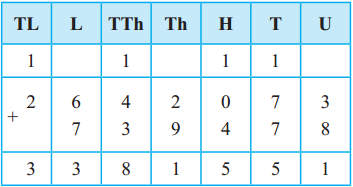Example (2)
26,42,073 + 7,39,478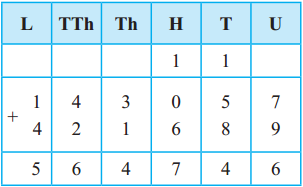Example (3)
3,12,469 + 758 + 24,092
3 1 2 4 6 9
+ 7 5 8
+ 2 4 0 9 2
____________
3 3 7 3 1 9
____________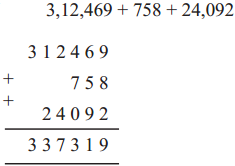Example (4)
64 + 409 + 5,13,728
6 4
+ 4 0 9
+ 5 1 3 7 2 8
_____________
5 1 4 2 0 1
_____________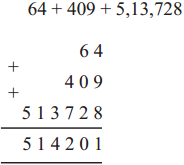In the examples 3 and 4, the numbers are carried over mentally.

Question 2.
(1)